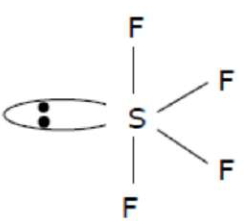# Which among the following species has unequal bond lengths?Question:

Which among the following species has unequal bond lengths?

1. $\mathrm{XeF}_{4}$

2. $\mathrm{SiF}_{4}$

3. $B F_{4}^{-}$

4. $\mathrm{SF}_{4}$

Correct Option: , 4

Solution:$\mathrm{Sp}^{3} \mathrm{~d}$ Hybridisation Sea-saw shape $\backslash \&$ axial bond length is more than equitorial bond length